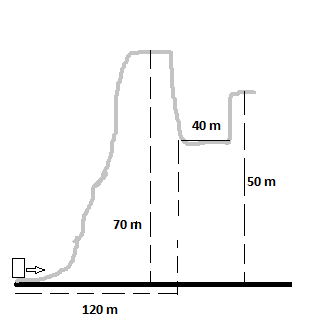# A 2.8 kg block slides over the smooth, icy hill shown in the figure below. The top of the hill is...

## Question:

A 2.8 kg block slides over the smooth, icy hill shown in the figure below. The top of the hill is horizontal and 70 m higher that its base. What minimum speed must the block have at the base of the hill so that it will not fall into the pit on the far side of the hill?## Projectile Motion:

When a particle is launched and acted upon by its weight alone, it is called a projectile. The horizontal and vertical ('up' positive) positions of the particle as a functions of time are (respectively):

{eq}x(t) = x_0 + \dot{x}_0 t\\ y(t) = y_0 + \dot{y}_0 t - \frac{1}{2}gt^2{/eq}

From the top of the hill, the particle will fly off as a projectile and will need to clear the pit to make it to the other side. The launch will happen horizontally. Thus, the time of flight, {eq}t_\star {/eq}, will be:

{eq}x(t_\star) = v t_\star \\ t_\star = \frac{40~\textrm{m}}{v} {/eq}

The minimum speed at the top of the ramp, however, will occur when {eq}y(t_\star) = -20~\textrm{m}{/eq}.

We will assume that the top of the hill is the origin of coordinates. Thus:

{eq}y(t_\star) = -\frac{1}{2}gt^2_\star\\ t_\star = \sqrt{\frac{2 |y(t_\star)|}{g}} = \sqrt{\frac{2 \cdot 20~\textrm{m}}{9.81~\textrm{m}~\textrm{s}^{-2}}} = 2.0~\textrm{s}{/eq}

We also need to recall:

{eq}x(t_\star) = v t_\star \\ v = \frac{x(t_\star)}{t_\star} = \frac{40~\textrm{m}}{2.02~\textrm{s}} = 20~\textrm{m}~\textrm{s}^{-1} {/eq}

Now, from conservation of energy, calling the bottom of the hill Point A (and origin of coordinates) and the top Point B, we find:

{eq}E_A = \frac{1}{2}mv^2_A = mgH + \frac{1}{2}mv^2_B = E_B\\ v_A = \sqrt{2 \frac{mgH + \frac{1}{2}mv^2_B}{m}} = \sqrt{2 \cdot \left(9.81~\textrm{m}~\textrm{s}^{-2} \cdot 70~\textrm{m} + \frac{1}{2} \cdot 20^2~\textrm{m}~\textrm{s}^{-2}\right)} = 30~\textrm{m}~\textrm/s {/eq}

The block must be traveling at a speed of 30 meters per second in order to not fall in the pit.